18 5 Coulombs Law 18 5 Coulombs Law

• Slides: 18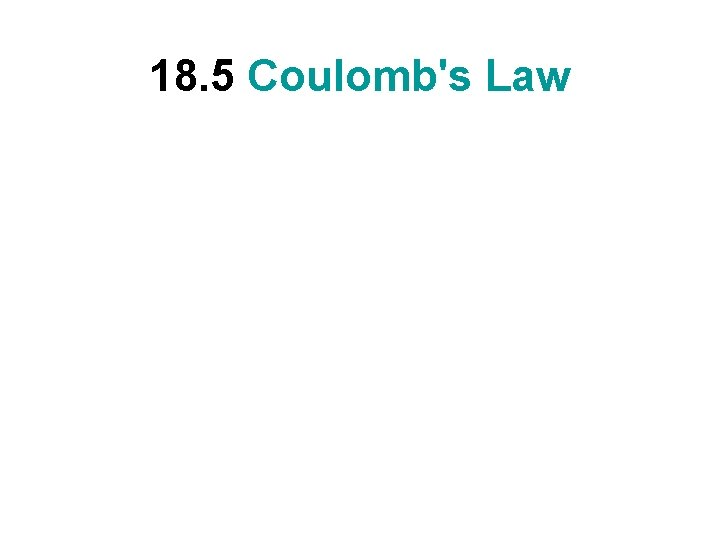18. 5 Coulomb's Law18. 5 Coulomb's Law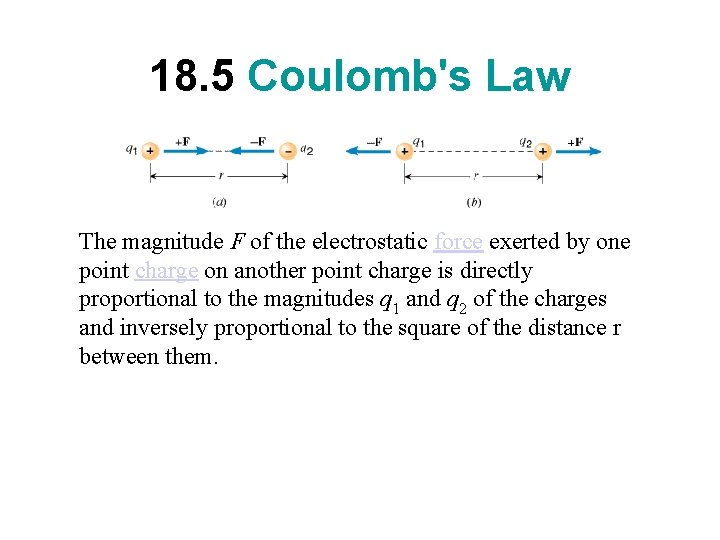18. 5 Coulomb's Law The magnitude F of the electrostatic force exerted by one point charge on another point charge is directly proportional to the magnitudes q 1 and q 2 of the charges and inversely proportional to the square of the distance r between them.18. 5 Coulomb's Law The magnitude F of the electrostatic force exerted by one point charge on another point charge is directly proportional to the magnitudes q 1 and q 2 of the charges and inversely proportional to the square of the distance r between them.18. 5 Coulomb's Law The proportionality constant k is known as Coulomb’s constant. It is given by, where ε 0 = 8. 85 10 -12 C 2/N. m 2, is called the permittivity of free space.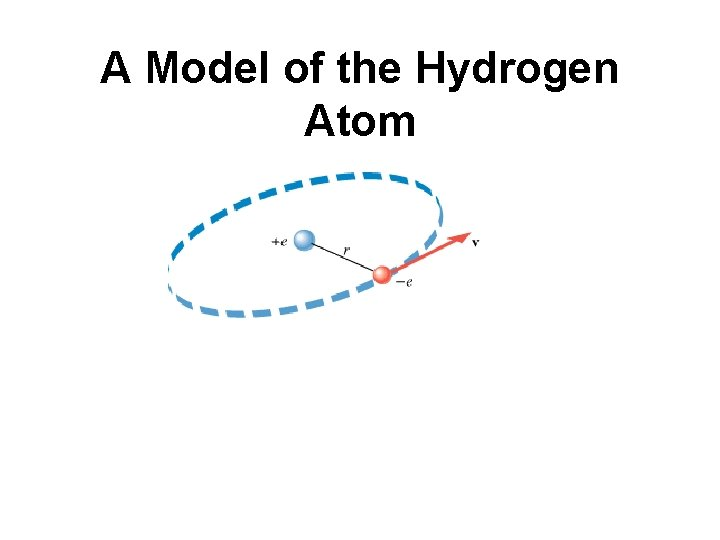A Model of the Hydrogen Atom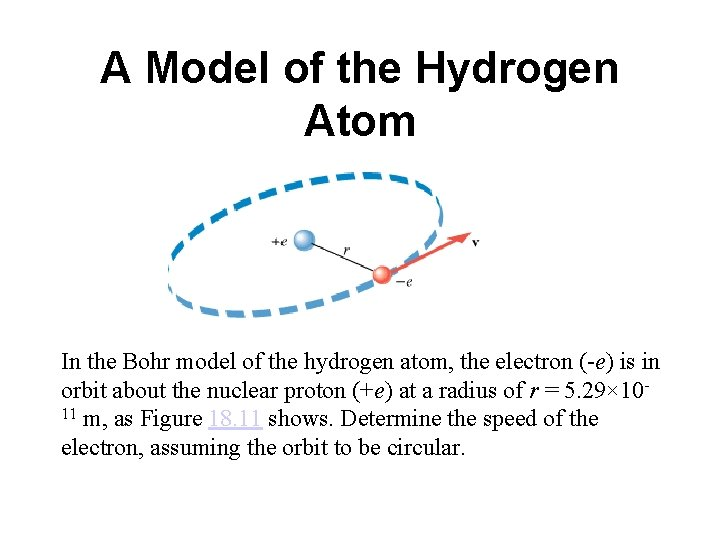A Model of the Hydrogen Atom In the Bohr model of the hydrogen atom, the electron (-e) is in orbit about the nuclear proton (+e) at a radius of r = 5. 29× 1011 m, as Figure 18. 11 shows. Determine the speed of the electron, assuming the orbit to be circular.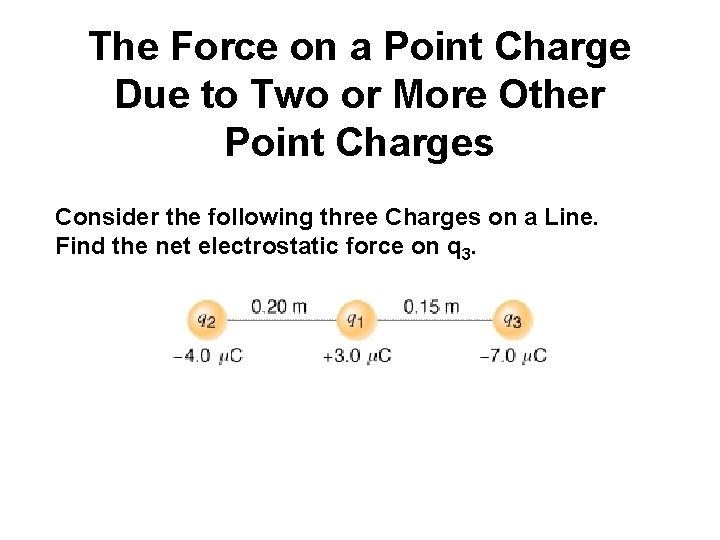The Force on a Point Charge Due to Two or More Other Point Charges Consider the following three Charges on a Line. Find the net electrostatic force on q 3.18. 6 The Electric Field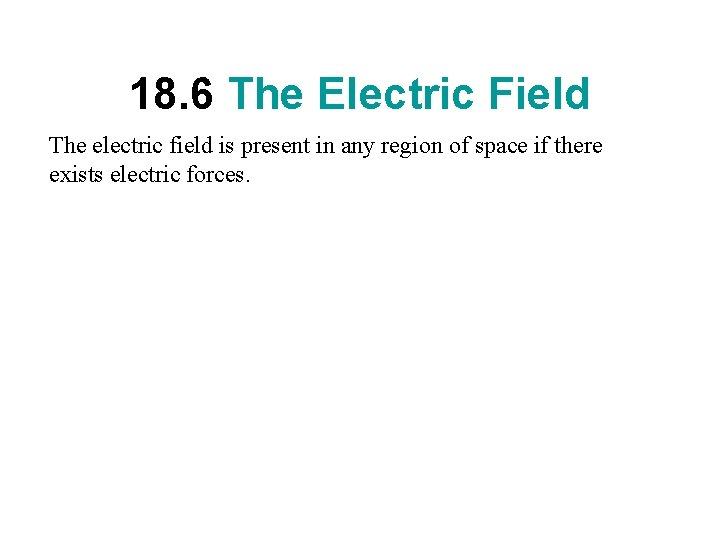18. 6 The Electric Field The electric field is present in any region of space if there exists electric forces.18. 6 The Electric Field The electric field is present in any region of space if there exists electric forces. These electric forces can be detected using a test charge. Test charges are theoretical positive charges that do not alter the electric field to be detected.18. 6 The Electric Field The electric field is present in any region of space if there exists electric forces. These electric forces can be detected using a test charge. Test charges are theoretical positive charges that do not alter the electric field to be detected. Electric field at a point in space is defined as the force per unit test charge placed at that point.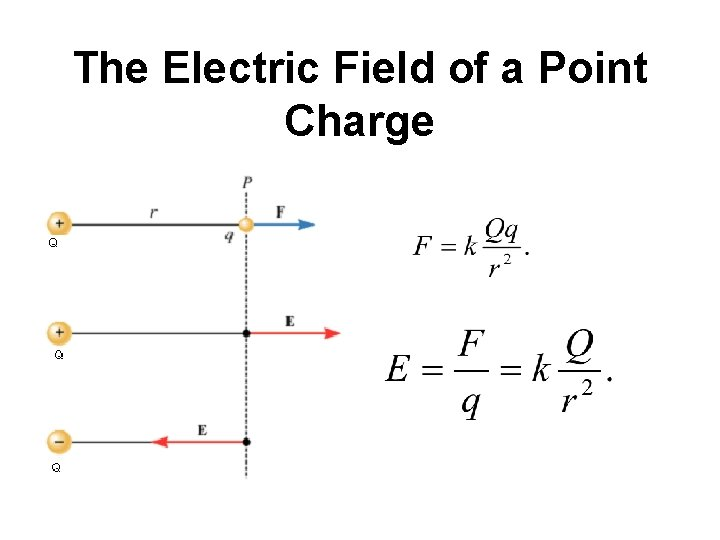The Electric Field of a Point ChargeProblem-29 Two charges are placed on the x axis. One charge (q 1 = +8. 5 m. C) is at x 1 = +3. 0 cm and the other (q 2 = -21 m. C) is at x 1 = +9. 0 cm. Find the net electric field (magnitude and direction) at x = 0 cm.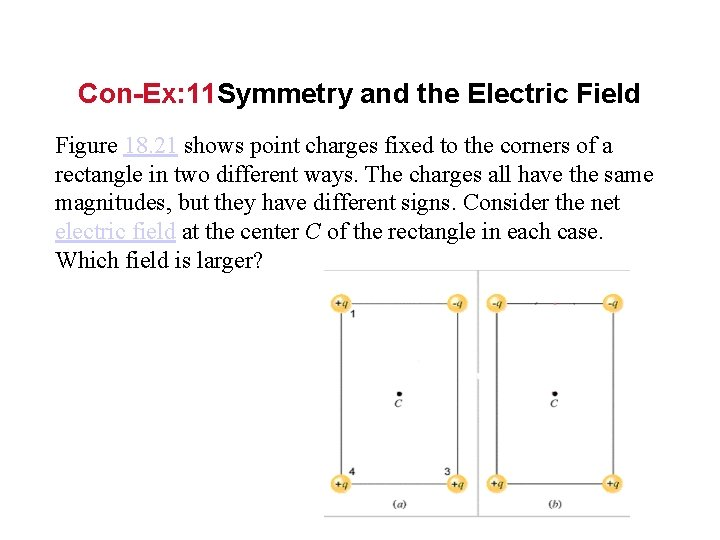Con-Ex: 11 Symmetry and the Electric Field Figure 18. 21 shows point charges fixed to the corners of a rectangle in two different ways. The charges all have the same magnitudes, but they have different signs. Consider the net electric field at the center C of the rectangle in each case. Which field is larger?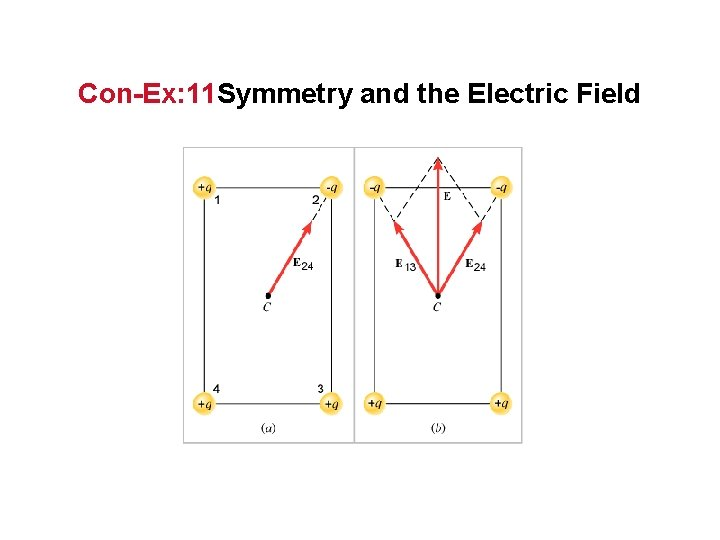Con-Ex: 11 Symmetry and the Electric Field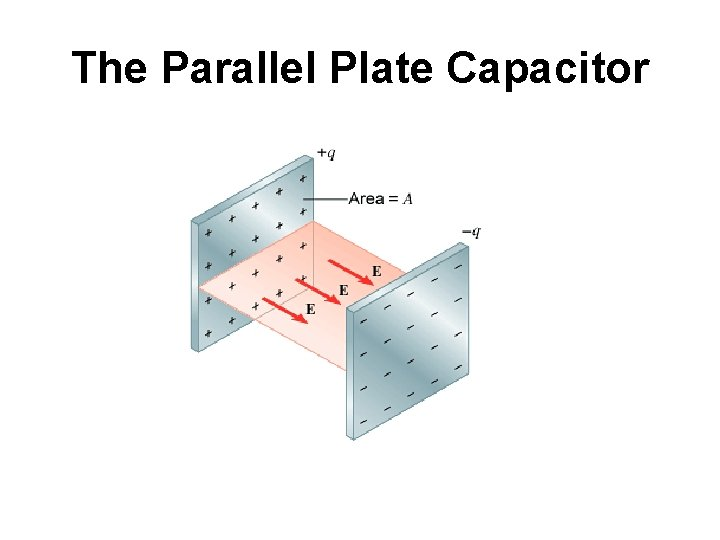The Parallel Plate CapacitorElectric field between two parallel plates